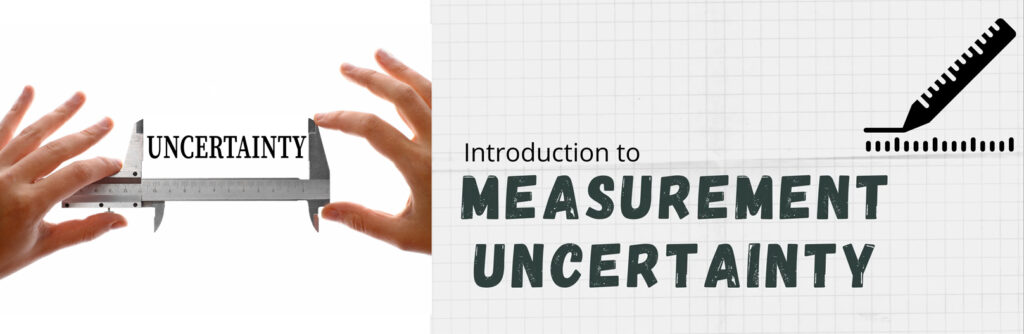## Measurement Uncertainty

Our labsTo describe purpose of Uncertainty in Calibration & Testing and explain the principles, processes and techniques used to evaluate Uncertainty in Measurement.

Explain the purpose, content and relationship of Uncertainty in Measurements and Lab Specific requirements

Interpret the Uncertainties in the context of improvements in the Test Methods & capabilities.

• Dimensional Laboratory Measurement Uncertainty
• Torque Laboratory Measurement Uncertainty
• Pressure Laboratory Measurement Uncertainty
• Acoustics Laboratory Measurement Uncertainty
• Mass Laboratory Measurement Uncertainty
• Volume Laboratory Measurement Uncertainty
• Density Laboratory Measurement Uncertainty
• Viscosity Laboratory Measurement Uncertainty
• Density Laboratory Measurement Uncertainty
• Viscosity Laboratory Measurement Uncertainty
• Thermal Laboratory Measurement Uncertainty
• Electro-Technical Laboratory Measurement Uncertainty
• Force Laboratory Measurement Uncertainty
• Hardness Laboratory Measurement Uncertainty
• Impact Laboratory Measurement Uncertainty
• Acceleration & Laboratory Measurement Uncertainty
• Fluid Flow Laboratory Measurement Uncertainty
• Optical Laboratory Measurement Uncertainty
• Mechanical Testing Laboratory Measurement Uncertainty